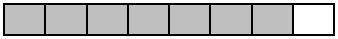# Fraction Operations

## Objective

Decompose fractions as a sum of unit fractions and as a sum of smaller fractions.

## Common Core Standards

### Core Standards

?

• 4.NF.B.3.B — Decompose a fraction into a sum of fractions with the same denominator in more than one way, recording each decomposition by an equation. Justify decompositions, e.g., by using a visual fraction model. Examples: 3/8 = 1/8 + 1/8 + 1/8 ; 3/8 = 1/8 + 2/8 ; 2 1/8 = 1 + 1 + 1/8 = 8/8 + 8/8 + 1/8.

?

• 3.NF.A.1

• 3.NF.A.2

## Criteria for Success

?

1. Decompose a fraction less than or equal to 1 into a sum of unit fractions, recording the decomposition with an equation.
2. Decompose a fraction less than or equal to 1 into a sum of fractions with the same denominator in more than one way, recording each decomposition with an equation.
3. Justify decompositions with a visual model, such as a tape diagram or number line.

## Tips for Teachers

?

• Throughout the unit, students will rely on tape diagrams and number lines to represent fraction operations. They won’t be formally introduced to the use of an area model to perform these operations until Grade 5, where they are particularly useful for helping to find a common unit when the units are unrelated to one another (e.g., 5 and 7, where they share no common factors). However, if students naturally choose to represent these computations with an area model or gravitate toward them, their use should not be discouraged.
• Before the Problem Set, you could have students play around with manipulatives to decompose fractions into a sum of unit fractions or non-unit fractions, similar to Joe Schwartz’s blog post “Building Towers”. Just make sure students are building fractions less than or equal to 1. If you don’t have fraction towers or even fraction tiles, you can create them from paper.

#### Fishtank Plus

• Problem Set
• Student Handout Editor
• Vocabulary Package

?

### Problem 1

1. Use the strips of paper given to you to fill in the following outline.What fraction of the whole outline is each piece that you used to fill it?

1. Come up with as many possible ways to fill the outline. Write all of the combinations of fractions you used to fill it.

### Problem 2

Decompose the fraction represented below into a sum of unit fractions and in at least one other way. Record the decompositions with equations.### Problem 3

1. Decompose the following fractions into a sum of unit fractions. Write an equation to record your decomposition.

i.   ${{3\over4}}$

ii.   ${{10\over10}}$

1. Find another way to decompose the fractions in part (a). Justify your decomposition with a tape diagram or number line.

## Discussion of Problem Set

?

• How else could you have decomposed any of the tape diagrams or number lines in #2? (You could choose to discuss which based on what’s best for your students.)
• Why does #3(a) start with “${1 =}$” as opposed to a fraction? What equivalent fraction could we use instead of $1$?
• What other decompositions could have been possible answers in #4?
• In #5, how would you record the fraction’s decomposition into unit fractions? How can you draw parentheses around a certain number of unit fractions to model another decomposition you wrote (e.g., $\left({1\over8}+{1\over8}\right)+\left({1\over8}+{1\over8}\right) + {1\over8}$ to model ${2\over8}+{2\over8}+{1\over8}$)?
• What relationship does the unit fraction have with the number of units in a whole?
• How did you decompose numbers in earlier grades? How is that similar to and different from how you decomposed fractions today?

?

Decompose the following fraction in three different ways, including as a sum of a unit of fractions. Record the decompositions with equations.

${{9\over10}}$

#### References

EngageNY Mathematics Grade 4 Mathematics > Module 5 > Topic A > Lesson 2Exit Ticket

Grade 4 Mathematics > Module 5 > Topic A > Lesson 2 of the New York State Common Core Mathematics Curriculum from EngageNY and Great Minds. © 2015 Great Minds. Licensed by EngageNY of the New York State Education Department under the CC BY-NC-SA 3.0 US license. Accessed Dec. 2, 2016, 5:15 p.m..

Modified by The Match Foundation, Inc.

?